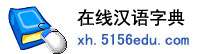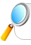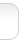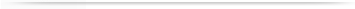汉字   按部首检索  按拼音检索李与 人与 主与 尹与 王与

 与字的男姓名 | 与字的女姓名 | 与开头的姓名 | 与结尾的姓名 | 更多带与的姓名
 与 (男) 与昕 (男) 子与 (男) 与点 (女) 与天 (男) 与龙 (男) 与弘 (男) 与彬 (男) 与非 (男) 与嵌 (女) 与辉 (男) 与涛 (男) 与国 (男) 与建 (男) 梦与 (男) 与任 (男) 与兴 (男) 与霆 (女) 倩与 (男) 与庭 (男) 与斌 (男) 与敏 (男) 与畅 (男) 与先 (男) 与皆 (男) 与敏 (女) 与炳 (男) 与才 (男) 与照 (男) 至与 (男) 与禄 (男) 与依 (男) 与涵 (男) 险与 (男) 与宏 (男) 与图 (男) 与恒 (男) 与春 (男) 与良 (男) 显与 (男) 与剑 (男) 与东 (男) 与琼 (女) 韦与 (女) 与强 (男) 景与 (男) 与凯 (男) 与钢 (男) 与铨 (女) 与聪 (男) 与忻 (女) 与振 (男) 与卓 (男) 与琴 (男) 与金 (男) 与波 (男) 与山 (男) 与华 (男) 与顺 (男) 与茂 (男) 与同 (女) 与 (女) 曜与 (女) 与华 (女) 与军 (男) 与礼 (男) 与月 (男) 与冲 (男) 与雄 (男) 辑与 (男) 与韶 (女) 与辰 (女) 与培 (女) 昊与 (男) 与周 (男) 善与 (男) 与文 (女) 与文 (男) 与阳 (男) 与意 (男) 正与 (男)

 版权所有　在线汉语字典   浙ICP备05019169号